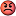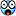1. ## Algebra help.

Can any of you check this out for me. i started getting back into math again and i kind of suck at it. This is a question out of one of my text books and they come up with a diffrent answer and i think they are wrong.

2x^3 - x^2 + y where x = -3 & y = 2

I come up with:

2(-3)^ 3 - (-3)^2 + 2

2(-27) - (-9) + 2

(-54) - (-9) + 2 = 61

they come up with

2(-3)^ 3 - (-3)^2 + 2

2(-27) - (9) + 2

-54 - 9 + 2 = -61

but wouldn't (-3^2) be (-9) not 9?

ThanksReply With Quote

2. hi
A negative number to the power of an even number returns a positive. So, -3^2 = 9, -3^3 = -27 and -3^4=81

2x^3 - x^2 + y where x = -3 & y = 2

2(-3^3) - (-3^2) + 2
2(-27) - (9) + 2
-54 - 9 + 2
= -61

Regards
StuartReply With Quote

3. Code:
```3 + (-3)  = 0

so as anything * 0 = 0

3 * (3 + -3) = 0 = (3 + -3) * -3

multiplying out
(3 * 3) + ( 3 * -3)  = ( 3 * -3) + ( -3 * -3)

and we can subtract ( 3 * -3) from both sides

(3 * 3) = ( -3  * -3)

(-3)^2  = 9```Reply With Quote

4. So the book was basically wrong.I didn't know that a negative number to the power of an even number returns a positive. Thanks that will come in handy.Reply With Quote

5. Originally posted by Dilenger4
So the book was basically wrong.I didn't know that a negative number to the power of an even number returns a positive. Thanks that will come in handy.LOL no, the book was basically right.. you were basically wrongReply With Quote

6. Beachbum don't state it like a formula that a negative number squared will give you a positive number. Explain him the logic.

Let's do it with 1

(+1) x (+1) = +1

(+1) x (-1) = -1

(-1) x (+1) = -1

(-1) x (-1) = +1

So you see that.. if you multiply a negative number 'a' to another number 'b' the resultant product 'c' has the opposite sign of 'b'.

There fore 1 x -1 x 1
can be processed as

1 x -1 = -1 which in turned multiplied by the third number 1

-1 x 1 = -1

like this you can see (-1)2 = -1 x -1 = +1

while (-1)3 = -1 x -1 x -1 = -1Reply With Quote

7. LOL no, the book was basically right.. you were basically wrong
How do you figure?(-3)^2 = -9 which i came up with. The book came up with 9.Reply With Quote

8. Originally posted by thinktank
Beachbum don't state it like a formula that a negative number squared will give you a positive number. Explain him the logic.
as Jamie would say ... pfffft!!Dilinger no that isnt true.. - 3 ^ 2 = -3 x -3 = 9
Look at Thinktank's post for explanation... and then feel free to say Bollox to him as well!!Reply With Quote

9. thinktank yes you are right. So i guess i could think of it this way.

When multiplying numbers with the same sign, multiply the absoulte value of each number. The product will always be positive whether the numbers are bolth positive or bolth negative

The same for numbers with diffrent signs but the result will always be negative.Reply With Quote

10.Reply With Quote

11. Sorry Dilinger but u are getting ur negatives mixed up

-3 ^ 3 = -27
2 x -27 = -54 so lets store that away

-3 ^ 2 = 9 (-3 x -3)

and finally +2

so the formula is now -54 - (9) + 2 = -61
The minus comes from the formula itself ie
2x^3 - x^2 + y
and not fromthe result of x^2

Regards
StuartReply With Quote

12. Originally posted by Dilenger4
So i was right on the 2(-27) - (-9) + 2 part because (-3)^2 does in fact equal -9 but i was wrong in calculating (-54) - (-9) + 2 = which equals -61 not 61 like i came up with.But how do we calculate (-54) - (-9) + 2 = ?I guess you are seriously weak in the fundamentals(-3)2 = -3 x -3 = +9

-54 - (-9) = -54 -1(-9) taking -1 inside the bracket
-54 + (-1 x -9)

-54 + 9 = -45

__________________

-54 -(-9) + 2 = -54 + (-1 x -9) + 2 = -54 + 9 + 2 = -54 + 11

= -43Reply With Quote

13. yes you are right (-54) - (-9) + 2 = -61 I guess the surgeon general was right smoking marijuana does burn brain cells.Reply With Quote

14. Originally posted by Dilenger4
yes you are right (-54) - (-9) + 2 = -61 I guess the surgeon general was right smoking marijuana does burn brain cells.damn u Dilenger!!!arrghhh

-54 - 9 + 2 = -61
-54 - (-9) + 2 = -43Reply With Quote

15. oh yeah right.*~snickerReply With Quote

16. Pffft you bunch of morons. Bollox to yiz!
Christ you're arguing over algebra... its not like art lads. There's a right way, and a wrong way.

*mutter*stuart*mutter*wrong way*mutter*Reply With Quote

17. No arguments here... just lots of help, compassion and brotherly love.I dont know much about algebra but i know what i likeReply With Quote

18. Originally posted by beachbum
i like just lots of brotherly lovejesus christ ya puff!Reply With Quote

19. I know what i like too. Jelly doughnuts from my local bakery.
yummyReply With Quote

20. we don't have jelly donuts in aus...

do you mean jam donuts?Reply With Quote

21. well here in the UK we call them doughnuts!Reply With Quote

####Posting Permissions

• You may not post new threads
• You may not post replies
• You may not post attachments
• You may not edit your posts
•